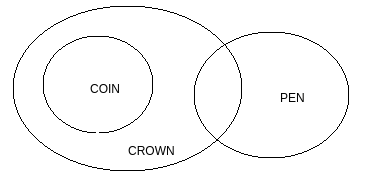# Directions : Given two statements, verify the conclusions and mark the answer as given below, Statements: I.          All coins are crows                    II.          Some crows are pens Conclusions: I.          No pen is coin                           II.          Some coins are pens   Option 1)   if only conclusion I follow.   Option 2)    If only conclusion II follows.   Option 3)    If either conclusion I or II follows.   Option 4)   If neither of the two conclusions follows.     Option 5)   If both conclusions follow.I. Not Follows

II. Not Follows

But these two are complimentary pairs so the answer would be Either.

Boost your Preparation for JEE Main 2021 with Personlized Coaching

Exams
Articles
Questions